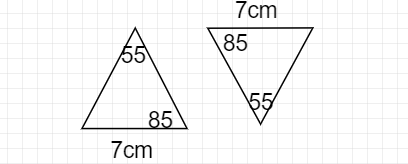"
">

# Prove that if two angles and one side of a triangle are equal to two angles and one side of another triangle. The triangles are congruent. Also check if the given pair of triangles are congruent?"

Given: Two angles and one side of a triangle are equal to two angles and one side of another triangle

To prove: The triangles are congruent

Solution:

The third angle in both triangles = 180° - 85° - 55° = 40°

In the two triangles

∠85° = ∠85°

7 cm = 7 cm

∠40°  = ∠40°

So ASA (Angle, included Side, Angle) congruence, the two triangles are CONGRUENT

Updated on: 10-Oct-2022

18 Views